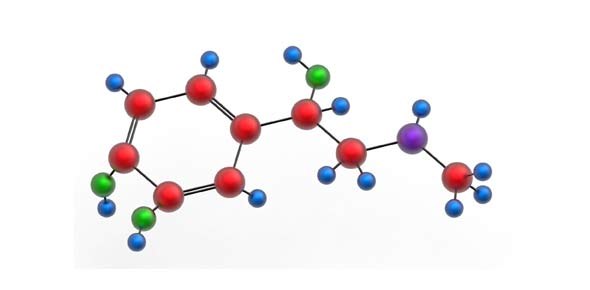# Carbon And Its Compounds Quiz: Trivia

15 Questions | Total Attempts: 4347Settings.

Related Topics
• 1.
• A.

(i)

• B.

(i) and (ii)

• C.

(i) , (ii) and (iii)

• D.

(i) and (iii)

• 2.
Which of the following compounds of carbon does not consist of ions?
• A.

CHCl3

• B.

CaCO3

• C.

NaHCO3

• D.

Ca2C

• 3.
Which of the following statements is not true? i. In the earth’s crust carbon is present in the form of minerals. ii. In the atmosphere very less amount of carbon is present. iii. In the earth’s crust very large amount of carbon is present.
• A.

(i)

• B.

(i) and (iii)

• C.

(iii)

• D.

(ii) and (iii)

• 4.
Which of the following structures correctly represents the electron dot structure of a chlorine molecule?
• A.
• B.
• C.
• D.
• 5.
Two compounds ‘A’ and ‘B’ are isomers. Which of the following statements, regarding A and B, is true? i. Both will have the same IUPAC name ii. Both will have the same structure iii. Both are hydrocarbons iv. Both have the same molecular formula
• A.

(i), (iii) and (iv)

• B.

(iii) and (iv)

• C.

(iv)

• D.

(iii)

• 6.
Which of the following compounds is an unsaturated hydrocarbon? i. Propane ii. Benzene iii. Acetylene
• A.

(i) and (iii)

• B.

(i) and (ii)

• C.

(iii)

• D.

(ii) and (iii)

• 7.
Which of the following bonds is very strong and hence very stable?
• A.

Carbon-Carbon bond

• B.

Carbon–Iodine bond

• C.

Carbon-Silicon bond

• D.

Carbon–Sulphur bond

• 8.
Read the given statements carefully and select the correct option for functional group i. The functional group can convert the uncreative unsaturated hydrocarbon into reactive one ii. The functional group can convert the uncreative saturated hydrocarbon into reactive one iii. The functional group decides the properties of inorganic compounds iv.  The functional group decides the properties of organic compounds or carbon compounds
• A.

(i) and (iii)

• B.

(i), (iii) and (iv)

• C.

(ii) and (iii)

• D.

(ii) and (iv)

• 9.
• A.

Alkenes are hydrocarbons

• B.

They are unsaturated compounds

• C.

They can consist of more than one double bond

• D.

The number of hydrogen atoms is always double the number of carbon atoms

• 10.
Which of the following is the molecular formula of cyclobutane?
• A.

C4H10

• B.

C 4H 6

• C.

C 4H 8

• D.

C 4H 4

• 11.
Which of the following statements about graphite and diamond is true?
• A.

They have the same crystal structure

• B.

They have the same degree of hardness

• C.

They have the same electrical conductivity

• D.

They can undergo the same chemical reactions

• 12.
While cooking, if the bottom of the vessel is getting blackened on the outside, it means that
• A.

The food is not cooked completely

• B.

The fuel is not burning completely

• C.

The fuel is wet

• D.

The fuel is burning completely

• 13.
Cation is formed when
• A.

Atom gains electrons

• B.

Atom loses electrons

• C.

Proton is lost by the atom

• D.

Atom shared by electrons

• 14.
Homologous series is a series of compounds in which
• A.

The same functional group substitutes for hydrogen in a carbon chain

• B.

Formulae of successive compounds differ by a –CH– unit

• C.

Representation is possible by any formula

• D.

Is collection of compounds with different functional groups

• 15.
• A.

Butene

• B.

Prop-1-ene

• C.

But-1-ene

• D.

Butyne